# JPEG2000 and specifying a target compression ratio

I was looking at some documentation yesterday and saw something that I had forgotten. When you write a JPEG2000 image file using imwrite, you can specify a desired compression ratio.

OK, that sounds fun. Let's try it with the peppers image.

rgb = imread('peppers.png');
imshow(rgb)
title('Original image')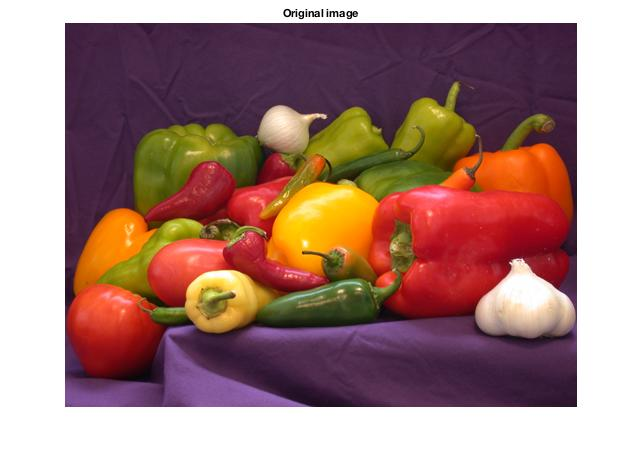imwrite(rgb,'peppers_10.j2k','CompressionRatio',10);
imshow('peppers_10.j2k')
title('Target compression ratio: 10')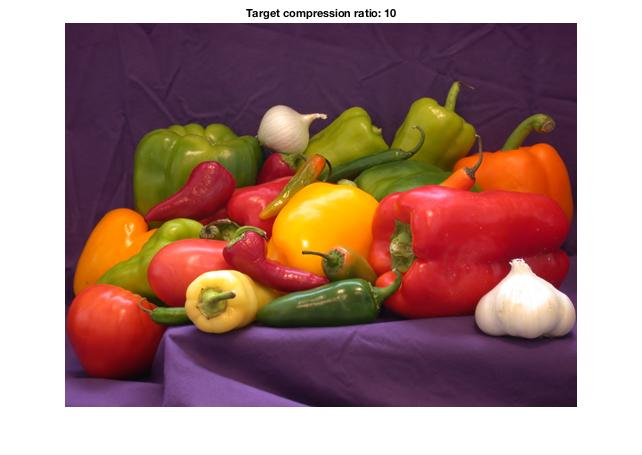That looks the same.

Hold on, though. Let's make sure we agree on what compression ratio means. And while we're at it, let's check the output file to verify the actual ratio.

The in-memory storage format for this image is 3 color values for each pixel, and each color value is stored using 1 byte. So the total number of in-memory bytes to represent the image is the number of rows times the number of columns times 3.

size(rgb)

ans =

384   512     3


num_mem_bytes = prod(ans)

num_mem_bytes =

589824



Now let's figure out the size of the JPEG2000 file we just created.

s = dir('peppers_10.j2k');
num_file_bytes = s.bytes

num_file_bytes =

58384



The compression ratio is the ratio of those two numbers.

r = num_mem_bytes / num_file_bytes

r =

10.1025



That's pretty close to the specified target.

Let's compress the image by a factor of 20.

imwrite(rgb,'peppers_20.j2k','CompressionRatio',20)
imshow('peppers_20.j2k')
title('Target compression ratio: 20')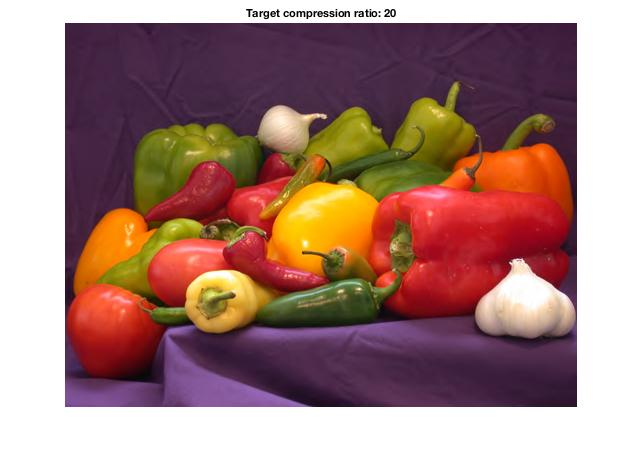I'm still seeing very little difference. Let's dial it all the way up to 100.

imwrite(rgb,'peppers_100.j2k','CompressionRatio',100)
imshow('peppers_100.j2k')
title('Target compression ratio: 100')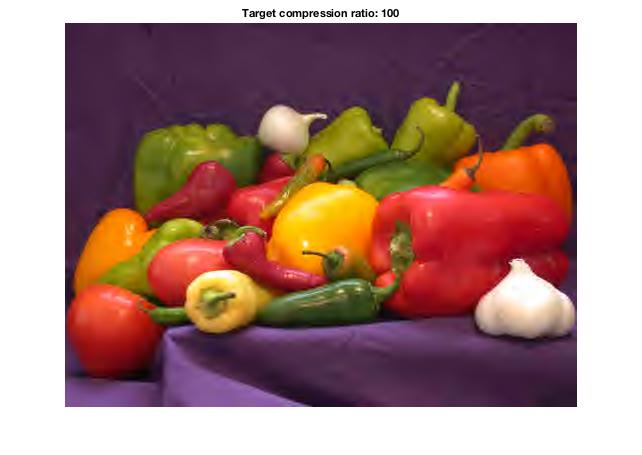Now that's getting pretty bad. Let's zoom into a region and compare more closely.

subplot(1,2,1)
imshow(rgb)
xlim([310 440])
ylim([30 130])
title('Original')

subplot(1,2,2)
imshow('peppers_100.j2k')
xlim([310 440])
ylim([30 130])
title('Target compression ratio: 100')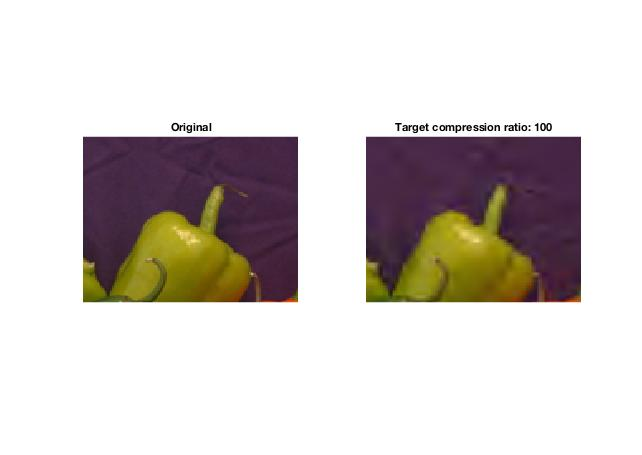How extreme can we get? Let's try 1000.

imwrite(rgb,'peppers_1000.j2k','CompressionRatio',1000)
clf
imshow('peppers_1000.j2k')
title('Target compression ratio: 1000')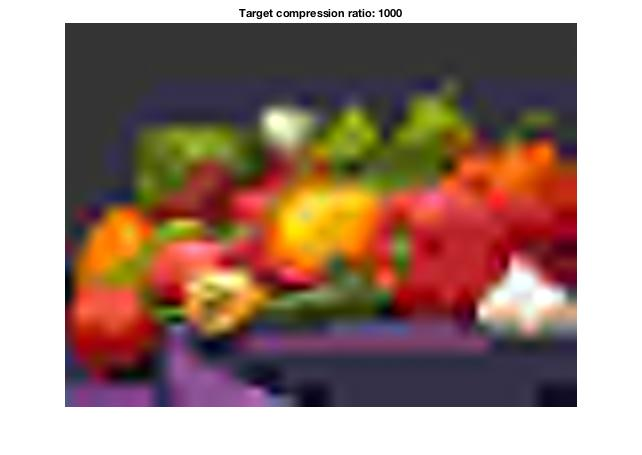That's pretty ugly. But how big is the file? Did we get close to the target?

s = dir('peppers_1000.j2k');
s.bytes

ans =

545


num_mem_bytes / s.bytes

ans =

1.0822e+03



That last image is stored using just 545 bytes! That's a compression ratio of about 1082.

I know JPEG2000 is used in some medical imaging systems, and I have also heard that the Library of Congress uses it.